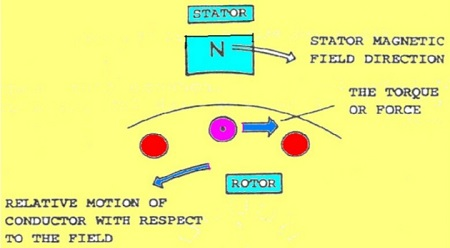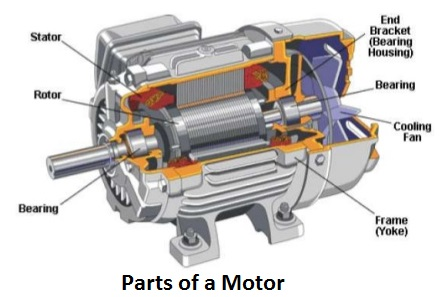# Introduction To Induction & AC Electric Motor | Working Principle & Types

An induction motor is an AC electric motor in which the electric current in the rotor of electric motor needed to produce torque is obtained by electromagnetic induction from the magnetic field of the stator winding. One of the most common electrical motor used in most applications is known as Induction Motor. Most commonly used motors are Three-phase and single phase induction motors. Induction electric motor is also called asynchronous motor because it runs at a speed less than synchronous speed which is the speed of rotation of the magnetic field in a rotary machine and it depends upon the frequency and number of poles of the motor.

## Working Principle of Induction Motor / Synchronous Motor

When ac supply is given to the stator winding of induction motor, the alternating current starts flowing through the stator or main winding. This alternating current produces an alternating flux called main flux. This main flux also links with the rotor conductors and hence cut the rotor conductor. Induction electric motors work under the Faraday’s law of electromagnetic induction.

According to the Faraday’s law of electromagnetic induction, emf gets induced in the rotor. As the rotor circuit is closed one so the current starts flowing in the rotor. This currents called the rotor current. This rotor current produces its own flux called rotor flux. Since this flux is produced due to induction principle so, the motor working on this principle got its name as induction motor. Now there are two fluxes one is main flux and another is called rotor flux. These two fluxes produce the desired torque which is required by the motor to rotate.  This is the fundamental operating principle of transformers, inductors, and many types of electrical motors, generators and solenoidsIn induction motor always runs at a speed less than synchronous speed because the rotating magnetic field which is produced in the stator will generate flux in the rotor which will make the rotor to rotate, but due to the lagging of flux current in the rotor with flux current in the stator, the rotor will never reach to its rotating magnetic field speed i.e. the synchronous speed. There are basically two types of induction electric motor that depend upon the input supply – single phase induction motor and three phase induction motor.

Slip of Of Induction Motor / Synchronous Motor

Induction motor rotor always rotate at a speed less than synchronous speed. The difference between the flux (Ns) and the rotor speed (N) is called slip.

% Slip = (Ns – N) x 100 / N

Slip speed = Ns – N

### Different Types of Induction Motors

SINGLE PHASE INDUCTION MOTORS

• Split phase induction motors
• Capacitor start induction electrical motor
• Capacitor start capacitor run induction motor
• Shaded pole induction motor •

THREE PHASE INDUCTION MOTORS

• Squirrel cage induction electric motors
• Slip ring induction electric motor

#### Construction of Three Phase Induction Motor

The three phase induction motor is the most widely used electrical motor. Almost 80% of the mechanical power used by industries is provided by three phase induction electric motors because of its simple and rugged construction, low cost, good operating characteristics, absence of commutator and good speed regulation. In three phase induction electrical motor the power is transferred from stator to rotor winding through induction. The Induction type electrical motor is also called asynchronous motor as it runs at a speed other than the synchronous speed.

Like any other electrical motor induction motors also have two main parts namely rotor and stator.

Stator: As its name indicates stator is a stationary part of an electric motor. A stator winding is placed in the stator of induction motors and the three phase supply is given to it.

Rotor: The rotor is a rotating part of induction electric motor. The rotor is connected to the mechanical load through the shaft. The rotor of the three phase induction motors are further classified as Squirrel cage rotor, Slip ring rotor or wound rotor or phase wound rotor. Depending upon the type of rotor construction used the three phase induction motor are classified as: Squirrel cage induction motor, Slip ring induction motor or wound induction motor or phase wound induction motor.#### SPEED CONTROL OF INDUCTION MOTORS

The speed of an induction motor can be easily controlled by varying the frequency of the 3-phase supply. To maintain a constant (rated) flux density, the applied voltage must also be changed in the same proportion as the frequency (as dictated by Faraday’s law). This electric motor speed control method is known as Volts per Hz. Above rated speed, the applied voltage is usually kept constant at rated value; this operation is referred to as constant HP. At low frequencies (i.e. speeds), the voltage must be boosted in order to compensate for the effects of the stator resistance.

##### Application of Three Phase & Single Phase Motors

Three phase induction motor are the motors most frequently used in different industries. They are simple, rugged, low price, and easy to maintain. They run at essentially constant speed from zero to full load. The speed is frequency-dependent and consequently, these motor are not easily adapted to speed control. In three phase system, there are three single phase line with 120° phase difference. So the rotating magnetic field is having the same phase difference which will make the rotor to move. Beside that the single phase motor also dominant for domestic and low power level, this is because it is used in home appliances and portable machine tool. In general, they are employed when three-phase power is not available. The construction of single phase induction electric motor is almost similar to the squirrel cage three-phase induction motor except that in case of single phase induction electric motor, the stator has two windings instead of one-phase as compare to the single stator winding per-phase in three phase induction motor.